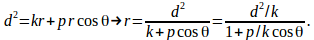## Proof That Trajectory of BodyAbout Another Body is a Conic Section

Suppose a body moves under the influence of a single mass producing a gravitational field. The equation of motion of the body is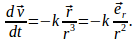Taking the cross product of this equation with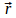gives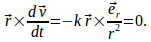Now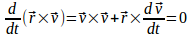since angular mometum is conserved.

Hence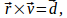a constant vector. We can write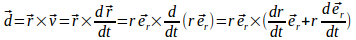Using the identity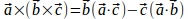gives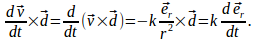Integrating this equation gives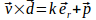and taking the dot product withgives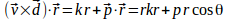where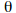is the angle between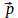and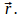Now use the identity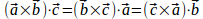to give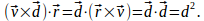Hence